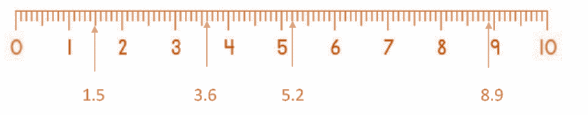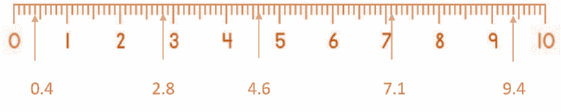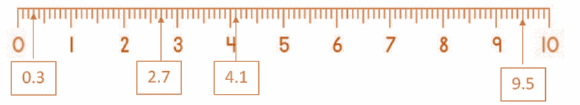# Decimals on a Number Line Worksheet 4th Grade (with answer key + PDF)

Another type of fraction is a decimal, in which a dot separates the whole number from the fractional portion. With the exception of how they are represented, fractions and decimals on a number line look very similar. We can perform precise calculations with the aid of decimals where the differences in minor values are crucial.

## What are the “Decimals on a Number Line Worksheet 4th Grade ”?

This worksheet will explore some of the decimals on a number line.

## What are Decimals on a Number Line?

A decimal number denotes a portion of a whole. It is, in other words, the interval between two integers. The distance between them can be divided into tenths by using a number line from 0 to 1. When asked to represent 0.3 on a number line, we would place a dot on the third tenth.

## Instructions on how to use the “Decimals on a Number Line Worksheet 4th Grade”

Study the concept and examples given and try to solve the exercises below.

## Conclusion

On a number line, decimal numbers are represented using the same method as whole numbers. For decimal numbers, tenths are represented by equally dividing the adjacent whole numbers to represent them. The hundredths are represented by further dividing each of the tenths into equal intervals.

If you have any inquiries or feedback, please let us know.

## Decimals on a Number Line Worksheet 4th Grade (with answer key + PDF)

A dot is used to demarcate the whole number from the fractional portion in decimals, another type of fraction. Except for the representation method, the representation of decimals on a number line is very similar to that of fractions.

Representing Decimals on Number Line

Plotting decimal numbers on a number line is referred to as representing decimals on a number line. A dot is used to denote the separation of the integral and fractional parts of decimal numbers. It is possible for the integral part to be less than 0 or more than 0. The decimals are positioned between the integers on a number line.

How to Represent Decimals on Number Line?

On a number line, fractional representation and decimal representation are very similar. On a number line, the left side of 0 represents the negative region, and the right side represents the positive region. Let’s use the methods listed below to represent 0.3 on a number line.

Step 1: Mark 0 as the reference point on a number line.

Step II: The second step is to identify and mark the integers that the decimal lies between. Between 0 and 1 is 0.3.

Step III: We will now divide the integers 0 and 1 by 10 to complete this step.

Step IV: From this point on, we’ll take as many steps to the right of 0 as there are digits to the right of the decimal point. We will move three spaces to the right for 0.3.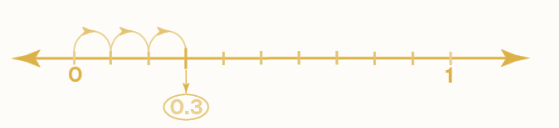Another example: We will represent 2.83 on the number line. We know that 2.83 lies between 2.8 and 2.9.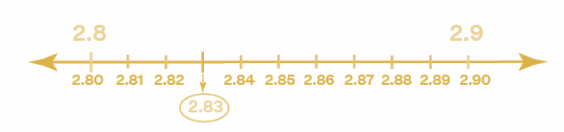## Worksheet

Decimal Number Lines

1. Write the missing decimal values on each of the arrows.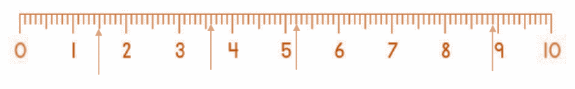1. Write the missing decimal values on each of the arrows.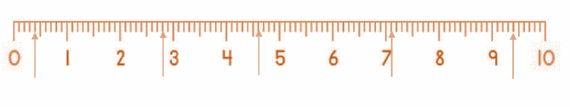1. Draw arrows to correctly place each number on the number line below.
1. Draw arrows to correctly place each number on the number line below.

1. Write the missing decimal values on each of the arrows.